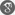##### Cite as:
N. Shobanadevi, J. Jagan Mohan, Analysis of Discrete Mittag - Leffler Functions, Int. J. Anal. Appl., 7 (2) (2015), 129-144.

#### Abstract

Discrete Mittag - Leffler functions play a major role in the development of the theory of discrete fractional calculus. In the present article, we analyze qualitative properties of discrete Mittag - Leffler functions and establish sufficient conditions for convergence, oscillation and summability of the infinite series associated with discrete Mittag - Leffler functions.

#### References

1. Agarwal, R.P., A propos d’une note de M.Pierre Humbert, C. R. Acad. Sci., 236 (1953), 2031 - 2032.2. Atsushi Nagai, Discrete Mittag - Leffler function and its applications, Publ. Res. Inst. Math. Sci., Kyoto Univ., 1302 (2003), 1 - 20.3. Bromwich, T.J., An Introduction to the Theory of Infinite Series, MacMillan, London, 1908.4. Butterworth, I.B., Infinite oscillation of alternating series, The Mathematical Gazette, 34 (1950), Number 310, 298 - 300.5. Elaydi, S., An Introduction to Difference Equations, Undergraduate Texts in Mathematics, 3rd Edition, Springer, New York, 2005.6. Ferhan M.Atici and Paul W.Eloe, Discrete fractional calculus with the nabla operator, Electron. J. Qual. Theory Differ. Equat., Special Edition I (2009), Number 13, 12 Pages (Electronic).7. Ferhan M.Atici and Paul W.Eloe, Gronwall’s inequality on discrete fractional calculus, Computers and Mathematics with Applications, 64 (2012), 3193 - 3200.8. Ferhan M.Atici and Paul W.Eloe, Linear systems of nabla fractional difference equations, Rocky Mountain Journal of Mathematics, 41 (2011), Number 2, 353 - 370.9. George A.Anastassiou, Nabla discrete fractional calculus and nabla inequalities, Mathematical and Computer Modelling, 51 (2010), 562 - 571.10. Gray, H.L. and Zhang, N.F., On a new definition of the fractional difference, Mathematics of Computaion, 50 (1988), Number 182, 513 - 529.11. Hardy, G.H., Divergent Series, Oxford Press, London, 1949.12. Hein, J., Mc Carthy, S., Gaswick, N., Mc Kain, B. and Spear, K., Laplace transforms for the nabla difference operator, Pan American Mathematical Journal, 21 (2011), Number 3, 79 - 96.13. Jagan Mohan, J. and Deekshitulu, G.V.S.R., Solutions of nabla fractional difference equations using N - transforms, Commun. Math. Stat., 2 (2014), 1 - 16.14. Jagan Mohan, J., Deekshitulu, G.V.S.R. and Shobanadevi, N., Stability of nonlinear nabla fractional difference equations using fixed point theorems, Italian Journal of Pure and Applied Mathematics, 32 (2014), 165 - 184.15. Jagan Mohan, J. and Shobanadevi, N., Stability of linear nabla fractional difference equations, Proceedings of the Jangjeon Mathematical Society, 17 (2014), Number 4, 651 - 657.16. Jagan Mohan, J., Variation of parameters for nabla fractional difference equations, Novi Sad J. Math., 44 (2014), Number 2, 149 - 159.17. Jagan Mohan Jonnalagadda, Solutions of perturbed linear nabla fractional difference equations, Differential Equations and Dynamical Systems, 22 (2014), Number 3, 281 - 292.18. James M.Hylop, Infinite Series, Interscience Publishers, London, 1959.19. Jan Cermak, Tomas Kisela and Ludek Nechvatal, Stability and asymptotic properties of a linear fractional difference equation, Advances in Difference Equations 2012 (2012), Article ID 122.20. Lloyd Leroy Smail, Some generalizations in the theory of summable divergent series, Dissertation, Columbia University, 1913.21. Miller, K.S. and Ross, B., Fractional difference calculus, Proceedings of the International Symposium on Univalent Functions, Fractional Calculus and Their Applications, 139 - 152, Nihon University, Koriyama, Japan, 1989.22. Mittag - Leffler, G.M., Sur la nouvelle fonction Eα(x), C. R. Acad. Sci. Paris, 137 (1903), 554 - 558.23. Nihan Acar, Ferhan M.Atici, Exponential functions of discrete fractional calculus, Applicable Analysis and Discrete Mathematics, 7 (2013), 343 - 353.24. Podlubny, I., Fractional Differential Equations, Academic Press, San Diego, 1999.25. Thabet Abdeljawad, Fahd Jarad and Dumitru Baleanu, A semigroup like property for discrete Mittag - Leffler functions, Advances in Difference Equations, 2012 (2012), Article ID 72.26. Thabet Abdeljawad and Ferhan M.Atici, On the definitions of nabla fractional operators, Abstract and Applied Analysis, 2012 (2012), Article ID 406757.